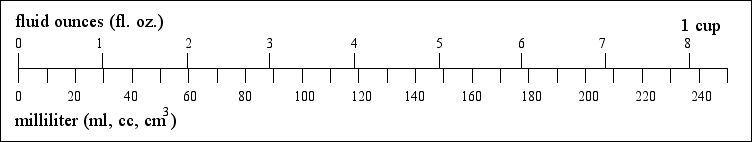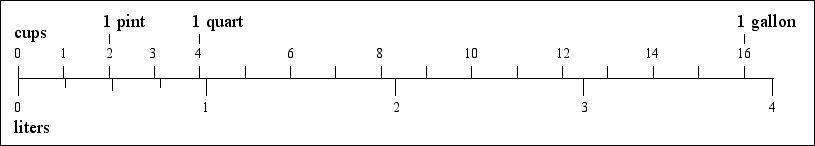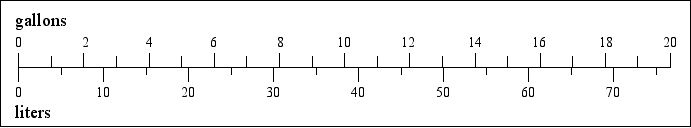# US and Metric Weights and Measures Compared

## Volume

### fluid ounces (fl. oz.) to milliliters (ml)1 fluid ounce = 29.573 milliliter 1 ml = 0.0338 fl. oz. 8 fl. oz = 1 cup = 236.6 ml

### cups to liters (L)1 pint = 2 cups = 0.473 liters 1 quart = 4 cups = 0.946 liters 1 gallon = 16 cups = 4 quarts = 3.785 liters (The old English fluid ounce was 28.4 ml and the UK gallon was 4.54 liters)

### gallons to liters1 gallon = 3.785 liters 1 liter = 0.264 gallon
Length inches to millimeters and inches to centimeters feet to meters and yards to meters miles to kilometers fluid ounces to milliliters, cups to liters and gallons to liters ounces to grams and pounds to kilograms Fahrenheit to Celsius

Marco Schuffelen - email